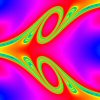# Chaotic ScatteringPlot of a cross section of the stable manifold of the chaotic invariant set from scattering off a potential of the form V(x,y)=(x^2)*(y^2)*exp[-(x^2+y^2)]. Created by taking a grid of initial conditions (initial x coordinate,initial velocity angle theta) at a given energy and integrating them forward. The color is proportional to the time the particle remains trapped. The stable manifold appears smooth and swirling along one direction with fractal structure transverse to that direction.